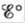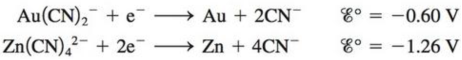Chapter 21, Problem 73AE

Chapter
Section
Textbook Problem

# Use standard reduction potentials to calculate, ΔG°, and K (at 298 K) for the reaction that is used in production of gold: 2 Au ( CN ) 2 − ( a q ) + Zn ( s ) → 2 Au ( s ) + Zn ( CN ) 4 2 − ( a q ) The relevant half-reactions areInterpretation Introduction

Interpretation: The values of Ecellο , ΔGο and K for the reaction that is used in the production of gold are to be calculated.

Concept introduction: Thermodynamics is associated with heat, temperature and its relation with energy and work. It helps us to predict whether a process will take place or not. The spontaneity of the process is determined by the entropy change it produces in the universe.

To determine: The values of Ecellο , ΔGο and K for the reaction that is used in the production of gold.

Explanation

Explanation

The given reaction is,

2Au(CN)2(aq)+Zn(s)2Au(s)+Zn(CN)42(aq)

The half reactions are,

Au(CN)2+eAu+2CNEο=0.60V (1)

Zn+4CNZn(CN)42+2eEο=1.26V (2)

The equation (1) is multiplied by 2 to balance the charges. Hence, the half reactions becomes,

2Au(CN)2+2e2Au+4CNEο=0.60V (3)

Zn+4CNZn(CN)42+2eEο=1.26V (4)

Equation (4) is subtracted from equation (3) to get the required reaction.

Therefore, the Ecellο for the given reaction is calculated as,

Ecellο=E(equation3)οE(equation4)οEcellο=(0.60)(1.26)Ecellο=0.60+1.26Ecellο=0.66V_

The value of ΔGο is calculated by the formula,

ΔGο=nFEcellο

Where,

• ΔGο is the standard Gibb’s free energy

### Still sussing out bartleby?

Check out a sample textbook solution.

See a sample solution

#### The Solution to Your Study Problems

Bartleby provides explanations to thousands of textbook problems written by our experts, many with advanced degrees!

Get Started

#### Find more solutions based on key concepts